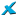! Please note that this is a snapshot of our old Bugzilla server, which is read only since May 29, 2020. Please go to gitlab.xfce.org for our new server !
Mousepad gets stuck in a busyloop
Status:
RESOLVED: DUPLICATE
Product:
Component:
General

Alistair Buxton 2015-05-23 20:33:46 CEST
```Mousepad sometimes gets stuck in a loop using 100% CPU and leaking around 4MB of memory per second. Here is a small bit of strace output:

Process 3497 attached
munmap(0x7f21e0261000, 19030)           = 0
munmap(0x7f21f3557000, 1)               = 0
access("/run", F_OK)                    = 0
stat("/run", {st_mode=S_IFDIR|0755, st_size=1040, ...}) = 0
access("/run/user", F_OK)               = 0
stat("/run/user", {st_mode=S_IFDIR|0755, st_size=60, ...}) = 0
access("/run/user/1000", F_OK)          = 0
stat("/run/user/1000", {st_mode=S_IFDIR|0700, st_size=200, ...}) = 0
access("/run/user/1000/dconf", F_OK)    = 0
stat("/run/user/1000/dconf", {st_mode=S_IFDIR|0700, st_size=40, ...}) = 0
open("/run/user/1000/dconf/user", O_RDWR|O_CREAT, 0600) = 11
pwrite(11, "\0", 1, 1)                  = 1
mmap(NULL, 1, PROT_READ, MAP_SHARED, 11, 0) = 0x7f21f3557000
close(11)                               = 0
open("/home/al/.config/dconf/user", O_RDONLY) = 11
fstat(11, {st_mode=S_IFREG|0664, st_size=19030, ...}) = 0
mmap(NULL, 19030, PROT_READ, MAP_PRIVATE, 11, 0) = 0x7f21e0261000
close(11)                               = 0
munmap(0x7f21e0261000, 19030)           = 0
munmap(0x7f21f3557000, 1)               = 0
access("/run", F_OK)                    = 0
stat("/run", {st_mode=S_IFDIR|0755, st_size=1040, ...}) = 0
access("/run/user", F_OK)               = 0
stat("/run/user", {st_mode=S_IFDIR|0755, st_size=60, ...}) = 0
access("/run/user/1000", F_OK)          = 0
stat("/run/user/1000", {st_mode=S_IFDIR|0700, st_size=200, ...}) = 0
access("/run/user/1000/dconf", F_OK)    = 0
stat("/run/user/1000/dconf", {st_mode=S_IFDIR|0700, st_size=40, ...}) = 0
open("/run/user/1000/dconf/user", O_RDWR|O_CREAT, 0600) = 11
pwrite(11, "\0", 1, 1)                  = 1
mmap(NULL, 1, PROT_READ, MAP_SHARED, 11, 0) = 0x7f21f3557000
close(11)                               = 0
open("/home/al/.config/dconf/user", O_RDONLY) = 11
fstat(11, {st_mode=S_IFREG|0664, st_size=19030, ...}) = 0
mmap(NULL, 19030, PROT_READ, MAP_PRIVATE, 11, 0) = 0x7f21e0261000
close(11)                               = 0
futex(0x7f21f41481f0, FUTEX_WAIT_PRIVATE, 2, NULL) = -1 EAGAIN (Resource temporarily unavailable)
futex(0x7f21f41481f0, FUTEX_WAKE_PRIVATE, 1) = 0
munmap(0x7f21e0261000, 19030)           = 0
munmap(0x7f21f3557000, 1)               = 0
access("/run", F_OK)                    = 0
stat("/run", {st_mode=S_IFDIR|0755, st_size=1040, ...}) = 0
access("/run/user", F_OK)               = 0
stat("/run/user", {st_mode=S_IFDIR|0755, st_size=60, ...}) = 0
access("/run/user/1000", F_OK)          = 0
stat("/run/user/1000", {st_mode=S_IFDIR|0700, st_size=200, ...}) = 0
access("/run/user/1000/dconf", F_OK)    = 0
stat("/run/user/1000/dconf", {st_mode=S_IFDIR|0700, st_size=40, ...}) = 0
open("/run/user/1000/dconf/user", O_RDWR|O_CREAT, 0600) = 11
pwrite(11, "\0", 1, 1)                  = 1
mmap(NULL, 1, PROT_READ, MAP_SHARED, 11, 0) = 0x7f21f3557000
close(11)                               = 0
open("/home/al/.config/dconf/user", O_RDONLY) = 11
fstat(11, {st_mode=S_IFREG|0664, st_size=19030, ...}) = 0
mmap(NULL, 19030, PROT_READ, MAP_PRIVATE, 11, 0) = 0x7f21e0261000
close(11)                               = 0
munmap(0x7f21e0261000, 19030)           = 0
munmap(0x7f21f3557000, 1)               = 0
access("/run", F_OK)                    = 0
stat("/run", {st_mode=S_IFDIR|0755, st_size=1040, ...}) = 0
access("/run/user", F_OK)               = 0
stat("/run/user", {st_mode=S_IFDIR|0755, st_size=60, ...}) = 0
access("/run/user/1000", F_OK)          = 0
stat("/run/user/1000", {st_mode=S_IFDIR|0700, st_size=200, ...}) = 0
access("/run/user/1000/dconf", F_OK)    = 0
stat("/run/user/1000/dconf", {st_mode=S_IFDIR|0700, st_size=40, ...}) = 0
open("/run/user/1000/dconf/user", O_RDWR|O_CREAT, 0600) = 11
pwrite(11, "\0", 1, 1)                  = 1
mmap(NULL, 1, PROT_READ, MAP_SHARED, 11, 0) = 0x7f21f3557000
close(11)                               = 0
open("/home/al/.config/dconf/user", O_RDONLY) = 11
fstat(11, {st_mode=S_IFREG|0664, st_size=19030, ...}) = 0
mmap(NULL, 19030, PROT_READ, MAP_PRIVATE, 11, 0) = 0x7f21e0261000
close(11)                               = 0
futex(0x7f21f41481f0, FUTEX_WAIT_PRIVATE, 2, NULL) = 0
futex(0x7f21f41481f0, FUTEX_WAKE_PRIVATE, 1) = 0
futex(0x7f21f4148300, FUTEX_WAKE_PRIVATE, 1) = 1
futex(0x7f21f41056d0, FUTEX_WAIT_PRIVATE, 2, NULL) = -1 EAGAIN (Resource temporarily unavailable)
futex(0x7f21f41056d0, FUTEX_WAKE_PRIVATE, 1) = 0
munmap(0x7f21e0261000, 19030)           = 0
munmap(0x7f21f3557000, 1)               = 0
access("/run", F_OK)                    = 0
stat("/run", {st_mode=S_IFDIR|0755, st_size=1040, ...}) = 0
access("/run/user", F_OK)               = 0
stat("/run/user", {st_mode=S_IFDIR|0755, st_size=60, ...}) = 0
access("/run/user/1000", F_OK)          = 0
stat("/run/user/1000", {st_mode=S_IFDIR|0700, st_size=200, ...}) = 0
access("/run/user/1000/dconf", F_OK)    = 0
stat("/run/user/1000/dconf", {st_mode=S_IFDIR|0700, st_size=40, ...}) = 0
open("/run/user/1000/dconf/user", O_RDWR|O_CREAT, 0600) = 11
pwrite(11, "\0", 1, 1)                  = 1
mmap(NULL, 1, PROT_READ, MAP_SHARED, 11, 0) = 0x7f21f3557000
close(11)                               = 0
open("/home/al/.config/dconf/user", O_RDONLY) = 11
fstat(11, {st_mode=S_IFREG|0664, st_size=19030, ...}) = 0
mmap(NULL, 19030, PROT_READ, MAP_PRIVATE, 11, 0) = 0x7f21e0261000
close(11)                               = 0
futex(0x7f21f4148300, FUTEX_WAKE_PRIVATE, 1) = 0
munmap(0x7f21e0261000, 19030)           = 0

Strace output grew to 20000 lines in a couple of seconds with nothing but this over and over.```
Andre Miranda2015-05-28 15:49:42 CEST
`Is there a pattern to reproduce this or is it totally random?`
Matthew Brush2015-06-03 02:59:47 CEST
```The only time I experienced something like this was when using the rectangular selection feature.

Does it happen when built against GTK3? The rectangular selection feature is disabled in the GTK3 build (since it uses API missing in GTK3), so maybe if it doesn't happen, it might be related.

I'm not sure what to make of the strace output but if, when it's stuck, the GLib mainloop is still pumping, it might explain the volume of output, and maybe the leakage is stuff that is meant to be freed when the loop is idle. That's just a total random guess though :)```
Matthew Brush2015-07-09 05:22:31 CEST
`@Alistair I think you mentioned on IRC that it might be when loading a really big single-line file?`
Alistair Buxton 2016-06-27 20:30:52 CEST
```This is a duplicate of 12413, where I have posted a patch.

*** This bug has been marked as a duplicate of bug 12413 ***```
Alistair Buxton 2016-06-27 20:32:26 CEST
```
*** This bug has been marked as a duplicate of bug 12134 ***```

### Bug #11927

Reported by:
Alistair Buxton
Reported on: 2015-05-23

Assignee:
Matthew Brush
CC List:
2 users

### Version

Target Milestone: